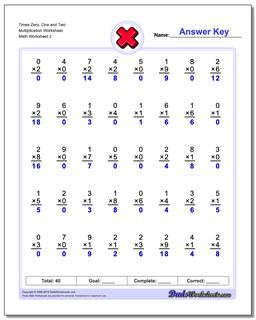One Dad. Four daughters. 9,268 worksheets... and counting!# Times Zero, One and Two Multiplication Worksheet (Second Worksheet)

PropertyValue
DescriptionTimes Zero, One and Two Multiplication Worksheet: Conventional times table math worksheets. These multiplication worksheets introduce math facts in order, as opposed to by fact families. If you grew up more than 20 years ago doing timed multiplication tests, these may look familiar.... (Second Worksheet)
Resource TypeWorksheet